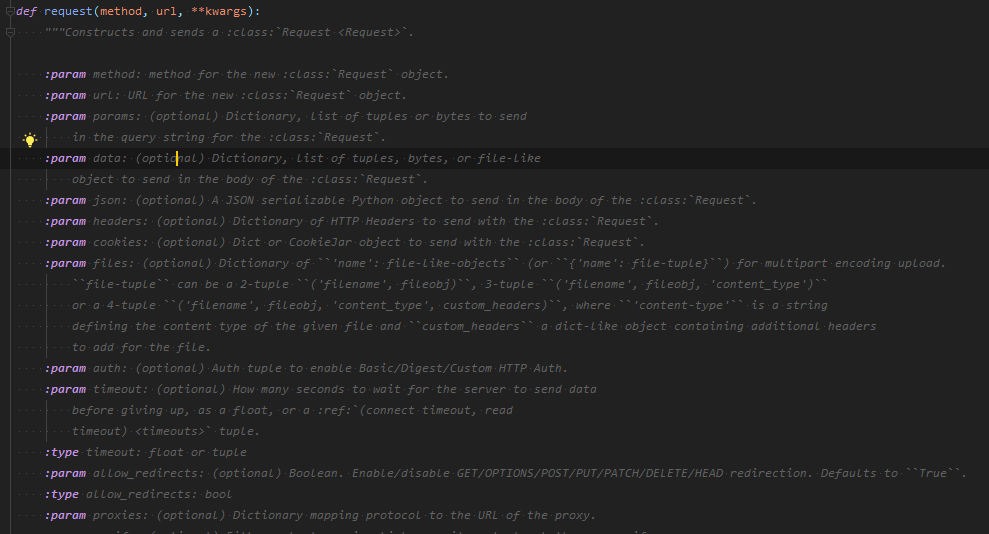# Python - 函数参数之必传参数、默认参数、可变参数、关键字参数的详细使用

## Python函数参数列表

• 必传参数：平时最常用的，必传确定数量的参数
• 默认参数：在调用函数时可以传也可以不传，如果不传将使用默认值
• 可变参数：可变长度参数
• 关键字参数：长度可变，但是需要以 key-value 形式传参

## 必传参数（位置参数）

def add(a, b):
return a + b

print(sum)

# 输出结果
3
• 传入的两个整数按照位置顺序依次赋给函数的参数 a 和 b，参数 a 和参数 b 被称为位置参数

### 传递的参数个数必须等于参数列表的数量

• 根据函数定义的参数位置来传递参数，要求传递的参数与函数定义的参数两者一一对应
• 如果 “传递的参数个数” 不等于 “函数定义的参数个数”，运行时会报错
# 错误栗子
return a + b

# 输出结果
E   TypeError: add() takes 2 positional arguments but 3 were given

## 默认参数

# 必传参数a,默认参数b
def test1(a, b=3):
print(a, b)

test1(0)
test1(0, 1)

#### 执行结果

0 3
0 1

### Python 字符串 index 函数

str.index(sub, start=None, end=None)

## 可变参数

### 为什么会需要可变参数

def add(a, b):
return a + b

add(1, 2)

def add3(a, b, c):
return a + b + c

add3(1, 2, 3)

#### 问题来了

• 那么，如果还需要一个 4 个数的求和函数，可以通过上述方式再加一个函数，但显然很麻烦
• 所以，可变参数诞生了

#### 可变参数的表示方式

def 函数(*args):
函数体
• 该函数被设定为能够接受可变数量的参数
• args 的类型是元组
• 调用该函数时，所有的参数被合并成一个元组
• 合并后的元组被赋值给 args，通过遍历 args 可以访问传递的参数

#### 求和的可变参数函数栗子

# 累加的可变参数函数
print(args)
sum = 0
for arg in args:
sum += arg
print('len = %d, sum = %d' % (len(args), sum))

# 输出结果
(1,)
len = 1, sum = 1
(1, 2)
len = 2, sum = 3
(1, 2, 3)
len = 3, sum = 6

### 混合栗子

def test2(num, num2=2, *args):
print(num, num2, args)

test2(1)
test2(1, 3)
test2(1, 3, 3, 3, 3, 3, 3)
test2(1, *(2, 3, 4, 5))
test2(1, *[2, 3, 4, 5])

#### 执行结果

1 2 ()
1 3 ()
1 3 (3, 3, 3, 3, 3)
1 2 (3, 4, 5)
1 2 (3, 4, 5)

### 知识点

• args是一个元组类型
• 可变参数可不传，也可以传很多个值
• *(2, 3, 4, 5) ，在元组or列表前面加一个 * ，代表将里面的每个元素独立出来，单独作为一个形参传进去，也称为元组解包

print(*(1, 2, 3, 4))
# 输出1 2 3 4

### 注意点一

# 可变参数
def myfun(a, *b):
print(a)
print(b)

myfun(a=1, 2, 3, 4)

# 输出结果
myfun(a=1, 2, 3, 4)
^
SyntaxError: positional argument follows keyword argument

### 注意点二

# 可变参数
def myfun(a, *b, c=None):
print(a)
print(b)
print(c)

myfun(1, 2, 3, 4)

# 输出结果
1
(2, 3, 4)
None 

c 输出了 None ，没有拿到参数值

#### 关键字传参解决这个问题

# 可变参数
def myfun(a, *b, c=None):
print(a)
print(b)
print(c)

myfun(1, 2, 3, c=4)

# 输出结果
1
(2, 3)
4

## 关键字参数

### 什么是关键字参数

Python 允许调用函数时，以 “参数名 = 参数值” 的形式传递参数

def add(a, b):
return a + b

add(a = 1, b = 2)    

"参数名 = 参数值" 形式的参数，例如 a = 1 和 b = 2，被称为关键字参数

### 包含关键字参数的函数语法格式

def 函数(**kwargs):
函数体 
• 该函数被设定为能够接受关键字参数
• kwargs 的类型是字典
• 调用该函数时，所有的关键字参数被合并成一个字典
• 合并后的字典被赋值给 kwargs，通过访问 kwargs 可以访问参数名和参数值

### 关键字参数栗子

# 关键字参数函数栗子
def create_full_name(**kwargs):
# 打印就是一个字典
print(kwargs)

name = kwargs.get("name")

print("only name is:", name)

# 方式一：通过 key=value 的方式传参

dict_ = {"name": "yy"}
# 方式二：传字典，然后加 ** 进行解包
create_full_name(**dict_)

# 输出结果
name is : 小菠萝  and password is : 123456

{'name': 'yy'}
only name is: yy

### 混合栗子

def test3(a, b=2, **kwargs):
print(a, b, kwargs)

test3(1)
test3(1, 3)
test3(1, **{"key": "value"})
test3(1, 3, **{"key": "value", "key1": "val", "key2": 'val2'})
test3(1, name="yy", key="value")
test3(1, 3, name="yy", key="value")
test3(1, name="yy", key="value",b=222222)
# test3(1, 22222,name="yy", key="value",b=222222) 会报错，因为第二个值已经传给默认参数b了

#### 执行结果

1 2 {}
1 3 {}
1 2 {'key': 'value'}
1 3 {'key': 'value', 'key1': 'val', 'key2': 'val2'}
1 2 {'name': 'yy', 'key': 'value'}
1 3 {'name': 'yy', 'key': 'value'}
1 222222 {'name': 'yy', 'key': 'value'}

### 知识点

• kwargs 是一个字典类型
• 关键字参数可传可不传
• 传值的时候需要传键值对，如果要传dict需要在前面加上 ** ，表示将这个dict的所有key-value当成独立的关键字参数（变成 key = value）传入到 kwargs，而修改 kwargs 不会影响原来的 dict
• 不用 dict 的话也可以直接 key=value 的写法，如果和默认参数重名，若前面没有传值的话，会当成默认参数传值；若有的话会直接报错
• 声明函数时，默认参数不可以放在关键字参数后面
• **dict ，也叫字典解包

### Python 库某个关键字函数栗子## 四种参数类型都有的函数

def test4(a, b=2, *args, **kwargs):
print(a, b, args, kwargs)

test4(1)
test4(1, 1)
test4(1, *(1, 2, 3, 3, 4,))
test4(1, *(1, 2, 3, 3, 4,), cc=123, c=123)

lists = ["a", "b"]
dicts = {"key": 123}
test4(1, *lists, **dicts)

### 执行结果

1 2 () {}
1 1 () {}
1 1 (2, 3, 3, 4) {}
1 1 (2, 3, 3, 4) {'cc': 123, 'c': 123}
1 a ('b',) {'key': 123}

## 拓展：Python 解包详解

https://www.cnblogs.com/poloyy/p/15096333.html

posted @ 2020-03-19 19:17  小菠萝测试笔记  阅读(4262)  评论(0编辑  收藏  举报